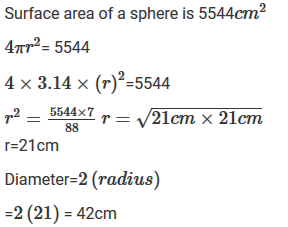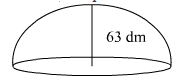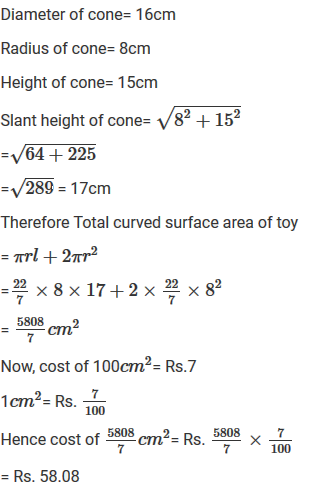#### Chapter 21 Surface Area and Volume of a  Sphere R.D. Sharma Solutions for Class 9th Exercise 21.1

Exercise 21.1

1. Find the surface area of a sphere of radius:
(i) 10.5 cm
(ii) 5.6 cm
(iii) 14 cm

Solution

Surface area= 4πr2
= 4×22/7×(10.5)2
= 1386cm2

Surface area= 4πr2
= 4×22/7×(5.6)2
= 394.24cm2

Surface area= 4πr2
= 4×22/7×(14)2
= 2464cm2

2. Find the surface area of a sphere of diameter.
(i) 14 cm
(ii) 21 cm
(iii) 3.5 cm

Solution

(i) Given Diameter = 14 cm
= 14/2 = 7cm
Surface area = 4πr2
= 4×22/7×(7)2
= 616cm2

(ii) Given Diameter = 21cm
= 2/12 = 10.5cm
Surface area = 4πr2
= 4×22/7×(10.5)2
= 1386cm2

(iii) Given diameter = 3.5cm
= 3.5/2 = 1.75cm
Surface area = 4πr2
= 4×22/7×(1.75)2
= 38.5cm

3. Find the total surface area of a hemisphere and a solid hemisphere each of radius 10cm (π =3.14)

Solution

The surface area of the hemisphere= 2πr2
= 2×3.14×(10)2
= 628cm2
The surface area of solid hemisphere=  2πr2
= 3×3.14×(10)2
= 942cm2

4. The surface area of a sphere is 5544 cm2, find its diameter .

Solution5. A hemispherical bowl made of brass has inner diameter 10.5 cm. Find the cost of tin-plating it on the inside at the rate of Rs 4 per 100 cm2.

Solution

Inner diameter of hemispherical bowl= 10.5cm
Surface area of hemispherical bowl= 2πr2
=2×3.14×(5.25)2
=173.25cm2
Cost of tin plating 100cm2 area= Rs.4
Cost of tin plating 173.25cm2 area= Rs. 4×173.25/100 = Rs. 6.93
Thus, the cost of tin plating the inner side of hemispherical bowl is Rs.6.93

6. The dome of a building is in the form of a hemisphere. Its radius is 63 dm. Find the cost of painting it at the rate of Rs 2 per sq. m.

SolutionInner surface area of dome= 2πr2
= 2×3.14×(6.3)2
= 249.48m2
Now, cost of 1m2= Rs.2
Therefore cost of 249.48m2 = Rs. (249.48×2) = Rs.498.96 .

7. Assuming the earth to be a sphere of radius 6370 km, how many square kilometres is area of the land, if three-fourth of the earth's surface is covered by water?

Solution

34th of earth surface is covered by water
Therefore 1/4th of earth surface is covered by land
Therefore Surface area covered by land= 1/4×4πr2
= 1/4×4×22/7×(6370)2
=127527400km2

8. A cylinder of same height and radius is placed on the top of a hemisphere. Find the curved surface area of the shape if the length of the shape be 7 cm.

SolutionGiven length of the shape= 7cm
But length = r+r
2r = 7cm
r = 3.5cm
Also; h = r
Total surface area of shape = 2πrh+2πr2
= 2πrr+2πr2
= 2πr2+2πr2
= 4×2/27×(3.5)2 = 154cm2

9. A wooden toy is in the form of a cone surmounted on a hemisphere. The diameter of the base of the cone is 16 cm and its height is 15 cm. Find the cost of painting the toy at Rs 7 per 100 cm2.

Solution10. A storage tank consists of a circular cylinder with a hemisphere adjoined on either end. If the external diameter of the cylinder by 1.4 m and its length be 8 m, find the cost of painting it on the outside at the rate of Rs. 10 per m2.

Solution

Diameter of a cylinder= 1.4m
Therefore radius of cylinder= 1.4/2 = 0.7m
Height of cylinder = 8m
Therefore surface area of tank= 2πrh+2πr2
= (2 × 2/27 × 0.7 × 8) + [2 × 22/7 × (0.7)2]
=176/5+77/25 = 38.28m2
Now cost of 1m2 = Rs.10
Therefore, cost of 38.28m2 = Rs. 382.80